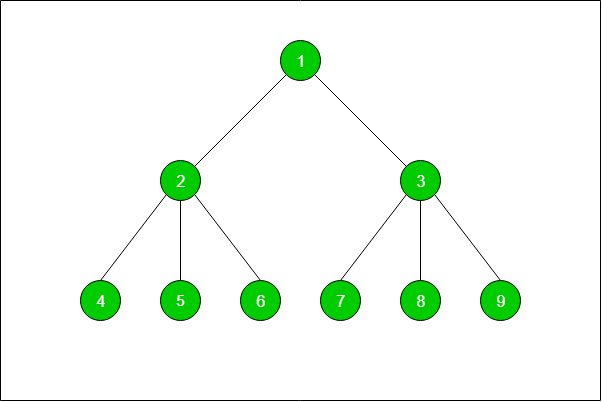# Print the path between any two nodes of a tree | DFS

Given a tree of distinct nodes N with N-1 edges and a pair of nodes P. The task is to find and print the path between the two given nodes of the tree using DFS.

```Input: N = 10
1
/    \
2      3
/ | \  / | \
4  5 6  7 8  9
Pair = {4, 8}
Output: 4 -> 2 -> 1 -> 3 -> 8

Input: N = 3
1
/  \
2    3
Pair = {2, 3}
Output:  2 -> 1 -> 3
```For example, in the above tree the path between nodes 5 and 3 is 5 -> 2 -> 1 -> 3.
Path between nodes 4 and 8 is 4 -> 2 -> 1 -> 3 -> 8.

## Recommended: Please try your approach on {IDE} first, before moving on to the solution.

Approach:

• The idea is to run DFS from the source node and push the traversed nodes into a stack till the destination node is traversed.
• Whenever backtracking occurs pop the node from the stack.

Note: There should be a path between the given pair of nodes.

Below is the implementation of the above approach:

## C++

 `// C++ implementation ` `#include ` `using` `namespace` `std; ` ` `  `// An utility function to add an edge in an ` `// undirected graph. ` `void` `addEdge(vector<``int``> v[], ` `             ``int` `x, ` `             ``int` `y) ` `{ ` `    ``v[x].push_back(y); ` `    ``v[y].push_back(x); ` `} ` ` `  `// A function to print the path between ` `// the given pair of nodes. ` `void` `printPath(vector<``int``> stack) ` `{ ` `    ``int` `i; ` `    ``for` `(i = 0; i < (``int``)stack.size() - 1; ` `         ``i++) { ` `        ``cout << stack[i] << ``" -> "``; ` `    ``} ` `    ``cout << stack[i]; ` `} ` ` `  `// An utility function to do ` `// DFS of graph recursively ` `// from a given vertex x. ` `void` `DFS(vector<``int``> v[], ` `         ``bool` `vis[], ` `         ``int` `x, ` `         ``int` `y, ` `         ``vector<``int``> stack) ` `{ ` `    ``stack.push_back(x); ` `    ``if` `(x == y) { ` ` `  `        ``// print the path and return on ` `        ``// reaching the destination node ` `        ``printPath(stack); ` `        ``return``; ` `    ``} ` `    ``vis[x] = ``true``; ` ` `  `    ``// if backtracking is taking place ` `    ``if` `(!v[x].empty()) { ` `        ``for` `(``int` `j = 0; j < v[x].size(); j++) { ` `            ``// if the node is not visited ` `            ``if` `(vis[v[x][j]] == ``false``) ` `                ``DFS(v, vis, v[x][j], y, stack); ` `        ``} ` `    ``} ` ` `  `    ``stack.pop_back(); ` `} ` ` `  `// A utility function to initialise ` `// visited for the node and call ` `// DFS function for a given vertex x. ` `void` `DFSCall(``int` `x, ` `             ``int` `y, ` `             ``vector<``int``> v[], ` `             ``int` `n, ` `             ``vector<``int``> stack) ` `{ ` `    ``// visited array ` `    ``bool` `vis[n + 1]; ` ` `  `    ``memset``(vis, ``false``, ``sizeof``(vis)); ` ` `  `    ``// DFS function call ` `    ``DFS(v, vis, x, y, stack); ` `} ` ` `  `// Driver Code ` `int` `main() ` `{ ` `    ``int` `n = 10; ` `    ``vector<``int``> v[n], stack; ` ` `  `    ``// Vertex numbers should be from 1 to 9. ` `    ``addEdge(v, 1, 2); ` `    ``addEdge(v, 1, 3); ` `    ``addEdge(v, 2, 4); ` `    ``addEdge(v, 2, 5); ` `    ``addEdge(v, 2, 6); ` `    ``addEdge(v, 3, 7); ` `    ``addEdge(v, 3, 8); ` `    ``addEdge(v, 3, 9); ` ` `  `    ``// Function Call ` `    ``DFSCall(4, 8, v, n, stack); ` ` `  `    ``return` `0; ` `} `

## Python3

 `# Python3 implementation of the above approach ` `v ``=` `[[] ``for` `i ``in` `range``(``100``)] ` ` `  `# An utility function to add an edge in an ` `# undirected graph. ` `def` `addEdge(x, y): ` `    ``v[x].append(y) ` `    ``v[y].append(x) ` ` `  `# A function to print the path between ` `# the given pair of nodes. ` `def` `printPath(stack): ` `    ``for` `i ``in` `range``(``len``(stack) ``-` `1``): ` `        ``print``(stack[i], end ``=` `" -> "``) ` `    ``print``(stack[``-``1``]) ` ` `  `# An utility function to do ` `# DFS of graph recursively ` `# from a given vertex x. ` `def` `DFS(vis, x, y, stack): ` `    ``stack.append(x) ` `    ``if` `(x ``=``=` `y): ` ` `  `        ``# print the path and return on ` `        ``# reaching the destination node ` `        ``printPath(stack) ` `        ``return` `    ``vis[x] ``=` `True` ` `  `    ``# if backtracking is taking place ` ` `  `    ``if` `(``len``(v[x]) > ``0``): ` `        ``for` `j ``in` `v[x]: ` `             `  `            ``# if the node is not visited ` `            ``if` `(vis[j] ``=``=` `False``): ` `                ``DFS(vis, j, y, stack) ` `                 `  `    ``del` `stack[``-``1``] ` ` `  `# A utility function to initialise ` `# visited for the node and call ` `# DFS function for a given vertex x. ` `def` `DFSCall(x, y, n, stack): ` `     `  `    ``# visited array ` `    ``vis ``=` `[``0` `for` `i ``in` `range``(n ``+` `1``)] ` ` `  `    ``#memset(vis, false, sizeof(vis)) ` ` `  `    ``# DFS function call ` `    ``DFS(vis, x, y, stack) ` ` `  `# Driver Code ` `n ``=` `10` `stack ``=` `[] ` ` `  `# Vertex numbers should be from 1 to 9. ` `addEdge(``1``, ``2``) ` `addEdge(``1``, ``3``) ` `addEdge(``2``, ``4``) ` `addEdge(``2``, ``5``) ` `addEdge(``2``, ``6``) ` `addEdge(``3``, ``7``) ` `addEdge(``3``, ``8``) ` `addEdge(``3``, ``9``) ` ` `  `# Function Call ` `DFSCall(``4``, ``8``, n, stack) ` `     `  `# This code is contributed by Mohit Kumar `

Output:

```4 -> 2 -> 1 -> 3 -> 8
```My Personal Notes arrow_drop_upCheck out this Author's contributed articles.

If you like GeeksforGeeks and would like to contribute, you can also write an article using contribute.geeksforgeeks.org or mail your article to contribute@geeksforgeeks.org. See your article appearing on the GeeksforGeeks main page and help other Geeks.

Please Improve this article if you find anything incorrect by clicking on the "Improve Article" button below.